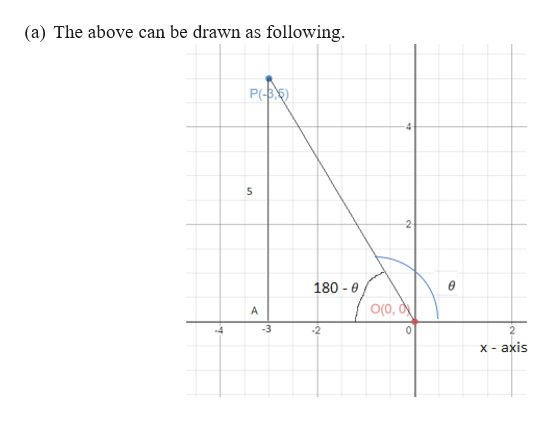# Consider a line segment connecting a point O (0,0) and point P (-3,5) as a terminal side which forms angle theta with x-axis.a. Draw a line segment above and angle theta on a x-y plane.b. Find the distance of the line segment OP.c. Find sine, cosine and tangent values of angle theta.

Question
1 views

Consider a line segment connecting a point O (0,0) and point P (-3,5) as a terminal side which forms angle theta with x-axis.

a. Draw a line segment above and angle theta on a x-y plane.

b. Find the distance of the line segment OP.

c. Find sine, cosine and tangent values of angle theta.

check_circle

Step 1

We consider a line segment connecting a point O (0,0) and point P (-...help_outlineImage Transcriptionclosefollowing (a) The above can be drawn as P(-B5 4 5 2 A 180-e O(O, d A -3 0 x axis fullscreen

### Want to see the full answer?

See Solution

#### Want to see this answer and more?

Solutions are written by subject experts who are available 24/7. Questions are typically answered within 1 hour.*

See Solution
*Response times may vary by subject and question.
Tagged in
MathTrigonometry

### Trigonometric Ratios